# Simplifying Higher-Order Plant Models

This example shows how to use Robust Control Toolbox™ to approximate high-order plant models by simpler, low-order models.

### Introduction

Robust Control Toolbox offers tools to deal with large models such as:

• High-Order Plants: Detailed first-principles or finite-element models of your plant tend to have high order. Often we want to simplify such models for simulation or control design purposes.

• High-Order Controllers: Robust control techniques often yield high-order controllers. This is common, for example, when we use frequency-weighting functions for shaping the open-loop response. We will want to simplify such controllers for implementation.

For control purposes, it is generally enough to have an accurate model near the crossover frequency. For simulation, it is enough to capture the essential dynamics in the frequency range of the excitation signals. This means that it is often possible to find low-order approximations of high-order models. Robust Control Toolbox offers a variety of model-reduction algorithms to best suit your requirements and your model characteristics.

### The Model Reduction Process

A model reduction task typically involves the following steps:

• Analyze the important characteristics of the model from its time or frequency-domain responses obtained from step or bode, for example.

• Determine an appropriate reduced order by plotting the Hankel singular values of the original model (hankelsv) to determine which modes (states) can be discarded without sacrificing the key characteristics.

• Choose a reduction algorithm. Some reduction methods available in the toolbox are: balancmr, bstmr, schurmr, hankelmr, and ncfmr

We can easily access these algorithms through the top-level interface reduce. The methods employ different measures of "closeness" between the original and reduced models. The choice is application-dependent. Let's try each of them to investigate their relative merits.

• Validation: We validate our results by comparing the dynamics of the reduced model to the original. We may need to adjust our reduction parameters (choice of model order, algorithm, error bounds etc.) if the results are not satisfactory.

### Example: A Model for Rigid Body Motion of a Building

In this example, we apply the reduction methods to a model of the building of the Los Angeles University Hospital. The model is taken from SLICOT Working Note 2002-2, "A collection of benchmark examples for model reduction of linear time invariant dynamical systems," by Y. Chahlaoui and P.V. Dooren. It has eight floors, each with three degrees of freedom - two displacements and one rotation. We represent the input-output relationship for any one of these displacements using a 48-state model, where each state represents a displacement or its rate of change (velocity).

Let's load the data for the example:

load buildingData.mat

### Examining the Plant Dynamics

Let's begin by analyzing the frequency response of the model:

bode(G) grid on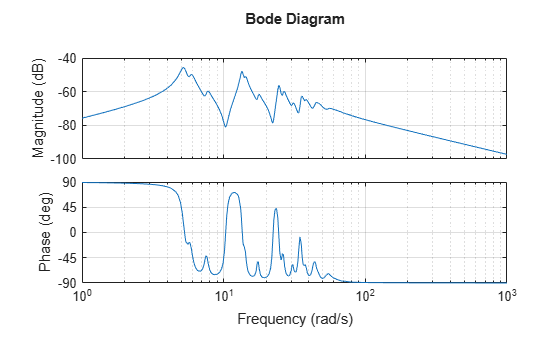Figure 1: Bode diagram to analyze the frequency response

As observed from the frequency response of the model, the essential dynamics of the system lie in the frequency range of 3 to 50 radians/second. The magnitude drops in both the very low and the high-frequency ranges. Our objective is to find a low-order model that preserves the information content in this frequency range to an acceptable level of accuracy.

### Computing Hankel Singular Values

To understand which states of the model can be safely discarded, look at the Hankel singular values of the model:

hsv_add = hankelsv(G); bar(hsv_add) title('Hankel Singular Values of the Model (G)'); xlabel('Number of States') ylabel('Singular Values (\sigma_i)') line([10.5 10.5],[0 1.5e-3],'Color','r','linestyle','--','linewidth',1) text(6,1.6e-3,'10 dominant states.')Figure 2: Hankel singular values of the model (G).

The Hankel singular value plot suggests that there are four dominant modes in this system. However, the contribution of the remaining modes is still significant. We'll draw the line at 10 states and discard the remaining ones to find a 10th-order reduced model Gr that best approximates the original system G.

### Performing Model Reduction Using an Additive Error Bound

The function reduce is the gateway to all model reduction routines available in the toolbox. We'll use the default, square-root balance truncation ('balancmr') option of reduce as the first step. This method uses an "additive" error bound for reduction, meaning that it tries to keep the absolute approximation error uniformly small across frequencies.

% Compute 10th-order reduced model (reduce uses balancmr method by default) [Gr_add,info_add] = reduce(G,10); % Now compare the original model G to the reduced model Gr_add bode(G,'b',Gr_add,'r') grid on title('Comparing Original (G) to the Reduced model (Gr\_add)') legend('G - 48-state original ','Gr\_add - 10-state reduced','location','northeast')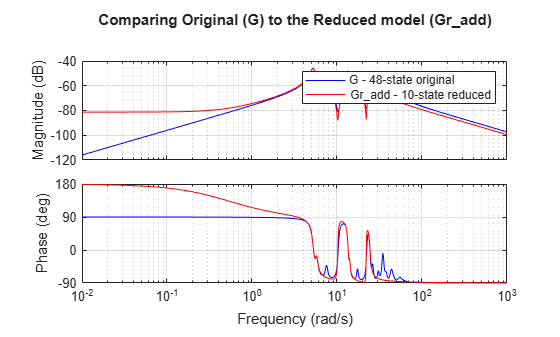Figure 3: Comparing original (G) to the reduced model (Gr_add)

### Performing Model Reduction Using a Multiplicative Error Bound

As seen from the Bode diagram in Figure 3, the reduced model captures the resonances below 30 rad/s quite well, but the match in the low frequency region (<2 rad/s) is poor. Also, the reduced model does not fully capture the dynamics in the 30-50 rad/s frequency range. A possible explanation for large errors at low frequencies is the relatively low gain of the model at these frequencies. Consequently, even large errors at these frequencies contribute little to the overall error.

To get around this problem, we can try a multiplicative-error method such as bstmr. This algorithm emphasizes relative errors rather than absolute ones. Because relative comparisons do not work when gains are close to zero, we need to add a minimum-gain threshold, for example by adding a feedthrough gain D to our original model. Assuming we are not concerned about errors at gains below -100 dB, we can set the feedthrough to 1e-5.

GG = G; GG.D = 1e-5;

Now, let's look at the singular values for multiplicative (relative) errors (using the 'mult' option of hankelsv)

hsv_mult = hankelsv(GG,'mult'); bar(hsv_mult) title('Multiplicative-Error Singular Values of the Model (G)'); xlabel('Number of States') ylabel('Singular Values (\sigma_i)')Figure 4: Multiplicative-error singular values of the model (G)

A 26th-order model looks promising, but for the sake of comparison to the previous result, let's stick to a 10th order reduction.

% Use bstmr algorithm option for model reduction [Gr_mult,info_mult] = reduce(GG,10,'algorithm','bst'); %now compare the original model G to the reduced model Gr_mult bode(G,Gr_add,Gr_mult,{1e-2,1e4}), grid on title('Comparing Original (G) to the Reduced models (Gr\_add and Gr\_mult)') legend('G - 48-state original ','Gr\_add (balancmr)','Gr\_mult (bstmr)','location','northeast')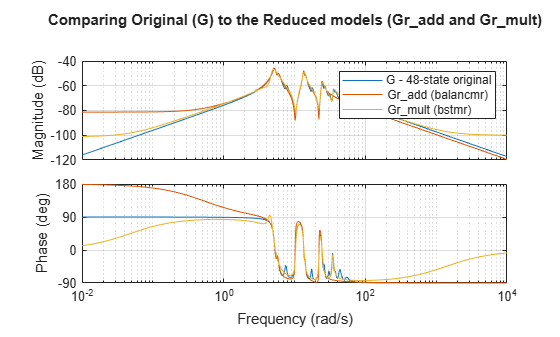Figure 5: Comparing original (G) to the reduced models (Gr_add and Gr_mult)

The fit between the original and the reduced models is much better with the multiplicative-error approach, even at low frequencies. We can confirm this by comparing the step responses:

step(G,Gr_add,Gr_mult,15) %step response until 15 seconds legend('G: 48-state original ','Gr\_add: 10-state (balancmr)','Gr\_mult: 10-state (bstmr)')Figure 6: Step responses of the three models

### Validating the Results

All algorithms provide bounds on the approximation error. For additive-error methods like balancmr, the approximation error is measured by the peak (maximum) gain of the error model G-Greduced across all frequencies. This peak gain is also known as the H-infinity norm of the error model. The error bound for additive-error algorithms looks like:

$‖G-G{r}_{add}{‖}_{\infty }\le 2\sum _{i=11}^{48}{\sigma }_{i}:=ErrorBound$

where the sum is over all discarded Hankel singular values of G (entries 11 through 48 of hsv_add). We can verify that this bound is satisfied by comparing the two sides of this inequality:

norm(G-Gr_add,inf) % actual error
ans = 6.0251e-04 
% theoretical bound (stored in the "ErrorBound" field of the "INFO" % struct returned by |reduce|) info_add.ErrorBound
ans = 0.0047 

For multiplicative-error methods such as bstmr, the approximation error is measured by the peak gain across frequency of the relative error model G\(G-Greduced). The error bound looks like

$‖{G}^{-1}\left(G-G{r}_{mult}\right){‖}_{\infty }\le \prod _{i=11}^{48}\left(1+2{\sigma }_{i}\left({\sigma }_{i}+\sqrt{1+{\sigma }_{i}^{2}}\right)\right)-1:=ErrorBound$

where the sum is over the discarded multiplicative Hankel singular values computed by hankelsv(G,'mult'). Again we can compare these bounds for the reduced model Gr_mult

norm(GG\(GG-Gr_mult),inf) % actual error
ans = 0.5949 
% Theoretical bound info_mult.ErrorBound
ans = 546.1730 

Plot the relative error for confirmation

bodemag(GG\(GG-Gr_mult),{1e-2,1e3}) grid on text(0.1,-50,'Peak Gain: -4.6 dB (59%) at 17.2 rad/s') title('Relative error between original model (G) and reduced model (Gr\_mult)')Figure 7: Relative error between original model (G) and reduced model (Gr_mult)

From the relative error plot above, there is up to 59% relative error at 17.2 rad/s, which may be more than we're willing to accept.

### Picking the Lowest Order Compatible with a Desired Accuracy Level

To improve the accuracy of Gr_mult, we'll need to increase the order. To achieve at least 5% relative accuracy, what is the lowest order we can get? The function reduce can automatically select the lowest-order model compatible with our desired level of accuracy.

% Specify a maximum of 5% approximation error [Gred,info] = reduce(GG,'ErrorType','mult','MaxError',0.05); size(Gred)
State-space model with 1 outputs, 1 inputs, and 35 states. 

The algorithm has picked a 34-state reduced model Gred. Compare the actual error with the theoretical bound:

norm(GG\(GG-Gred),inf)
ans = 0.0068 
info.ErrorBound
ans = 0.0342 

Look at the relative error magnitude as a function of frequency. Higher accuracy has been achieved at the expense of a larger model order (from 10 to 34). Note that the actual maximum error is 0.6%, much less than the 5% target. This discrepancy is a result of the function bstmr using the error bound rather than the actual error to select the order.

bodemag(GG\(GG-Gred),{1,1e3}) grid on text(5,-75,'Peak Gain: -43.3 dB (0.6%) at 73.8 rad/s') title('Relative error between original model (G) and reduced model (Gred)')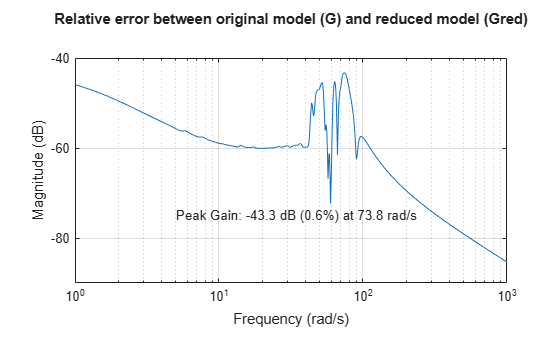Figure 8: Relative error between original model (G) and reduced model (Gred)

Compare the Bode responses

bode(G,Gred,{1e-2,1e4}) grid on legend('G - 48-state original','Gred - 34-state reduced')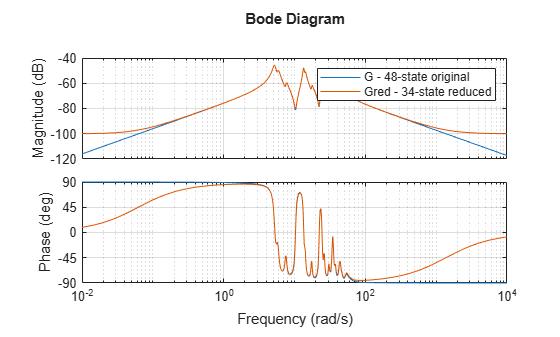Figure 9: Bode diagram of the 48-state original model and the 34-state reduced model

Finally, compare the step responses of the original and reduced models. They are virtually indistinguishable.

step(G,'b',Gred,'r--',15) %step response until 15 seconds legend('G: 48-state original ','Gred: 34-state (bstmr)') text(5,-4e-4,'Maximum relative error <0.05')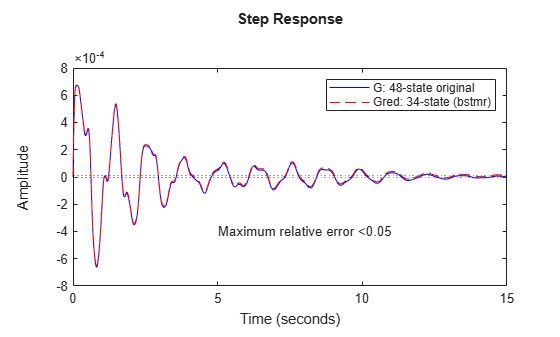Figure 10: Step response plot of the 48-state original model and the 34-state reduced model